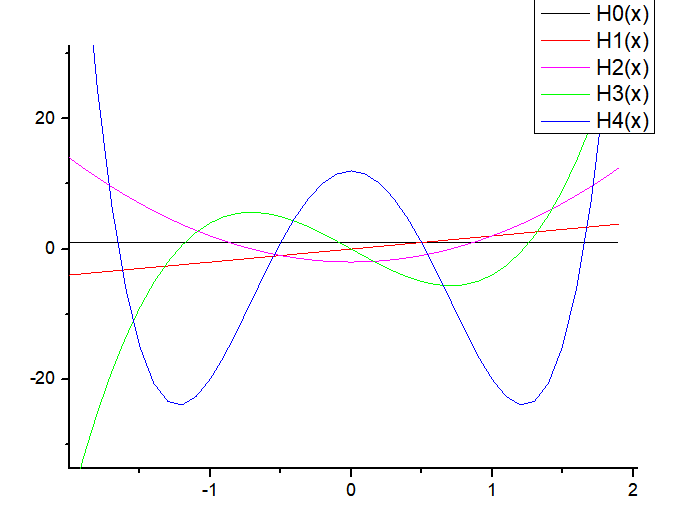# Hermite Polynomial – C PROGRAM

In this post I’m gonna show you how to calculate Hermite polynomials using three different techniques: using recurrence relations, series representations, and numerical integration.
The programs will calculate and plot the first few Hermite polynomials.

### Using Recurrence Relation

We will be using the following recurrence relation:$H_{n+1}(x)=2x H_n (x) - 2n H_{n-1}(x)$
We would need two more relations, that is the relations for 0th and 1st order Legendre polynomials:$H_0(x)=1$$H_1(x)=2x$
We will create a program that calculates the values of the Legendre polynomial at various x values and for different l and store these values in a txt file. Then just plot it using Gnuplot.
We will create two functions called ‘h0’ and ‘h1’, that contain the definition of respectively.
Then we will create a function ‘hn’ that will use the first two functions and recursion to find the value of Legendre polynomial for different x,n.
NOTE: I am using a slightly modified form of the recurrence relation. To get the form I am using, just replace n by n-1.

#### CODE:

#include<stdio.h>
#include<math.h>

double h0(double x){
return 1;
}

double h1(double x){
return 2*x;
}
//The following is a general function that returns the value of the Hermite Polynomial for any given x and n=0,1,2,3,...
double hn(double x,int n){
if(n==0){
return h0(x);
}
else if(n==1){
return h1(x);
}
else{
return 2*x*hn(x,n-1)-2*(n-1)*hn(x,n-2);
}
}
main(){
//We will create a data-file and store the values of first few Hermite polynomials for -1<x<5
FILE *fp=NULL;
//create data-file
fp=fopen("hermite1.txt","w");
double x;
//write the values of first 5 Hermite  polynomials to data-file
for(x=-2;x<=2;x=x+0.1){
fprintf(fp,"%lf\t%lf\t%lf\t%lf\t%lf\t%lf\n",x,hn(x,0),hn(x,1),hn(x,2),hn(x,3),hn(x,4));
}
}


#### OUTPUT:

The above program will create a data-file called legendre1.txt  and store the values of the first 5 Hermite polynomials for$-2\leq x \leq 2$. Now, you can just open the file and select the data and plot it using Excel, GnuPlot, Origin, etc.
For GnuPlot, the command is:First few Hermite polynomials using recurrence relation

### References:

http://mathworld.wolfram.com/HermitePolynomial.html[wpedon id="7041" align="center"]

## One thought on “Hermite Polynomial – C PROGRAM”

1. can you please tell me how can we plot the coherent state wavefunctions or probabilities using the above given function? I am not getting a proper Gaussian curve for the probability density of harmonic oscillator coherent states.